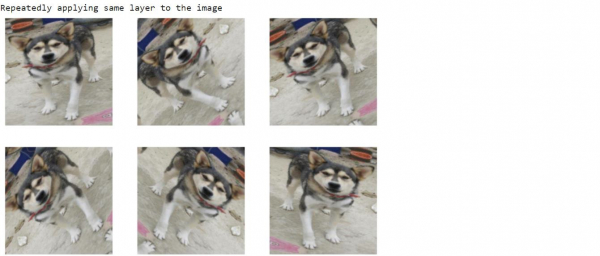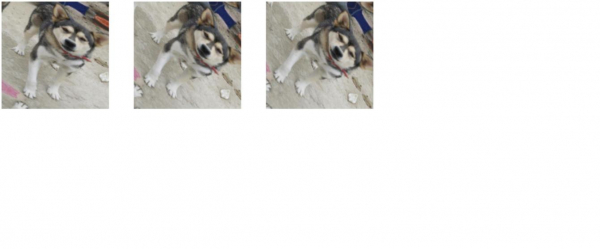# How can Tensorflow and pre-trained model be used to apply the same layer repeatedly to an image for data augmentation?

TensorflowServer Side ProgrammingProgramming

Tensorflow and the pre-trained model can be used to apply the same layer repeatedly on an image for data augmentation by calling the previously created ‘data_augmentation’ function on the image. This augmented image is also visualized on the console.

We will understand how to classify images of cats and dogs with the help of transfer learning from a pre-trained network.

The intuition behind transfer learning for image classification is, if a model is trained on a large and general dataset, this model can be used to effectively serve as a generic model for the visual world. It would have learned the feature maps, which means the user won’t have to start from scratch by training a large model on a large dataset.

We are using the Google Colaboratory to run the below code. Google Colab or Colaboratory helps run Python code over the browser and requires zero configuration and free access to GPUs (Graphical Processing Units). Colaboratory has been built on top of Jupyter Notebook.

## Example

print("Repeatedly applying same layer to the image")
for image, _ in train_dataset.take(1):
plt.figure(figsize=(10, 10))
first_image = image
for i in range(9):
ax = plt.subplot(3, 3, i + 1)
augmented_image = data_augmentation(tf.expand_dims(first_image, 0))
plt.imshow(augmented_image / 255)
plt.axis('off')

## Output## Explanation

• The data augmentation is odone by applying the layer to the image repeatedly until satisfactory results are obtained.

• The sample images are displayed on the console.